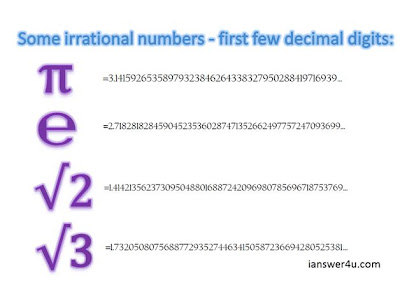# Irrational Numbers in Mathematics

### What are Irrational Numbers?

Irrational numbers are a subset of real numbers which are not rational numbers but can be represented on the number line. Any non-terminating and non-recurring decimal is an irrational number. An irrational number, unlike rational number, cannot be written in the form of p/q, where p and q are integers and q≠0. Between any two integers there are infinite irrational numbers. They are also called surds.
A non-recurring and non-terminating decimal is one which has one or more digits after the decimal point which goes on endlessly without any specific pattern or series which proves it is irrational. Pi is one of the famous examples of irrational numbers, and please note value of pi is not equal to 22/7.

### Examples of Irrational NumbersIrrational Numbers

### Operations on irrational numbers:

If we add, subtract, multiply or divide two irrationals, the result may be rational or irrational. For example:
√3 + √3 = 3.4641…., which is an irrational number,
√3 - √3 = 0, which is a rational number,
√3 × √3 = 3, which is a rational number and
√3 ÷ √3 = 1, which is a rational number.